Test: IPv4

# Test: IPv4

Test Description

## 10 Questions MCQ Test Computer Networks | Test: IPv4

Test: IPv4 for Computer Science Engineering (CSE) 2023 is part of Computer Networks preparation. The Test: IPv4 questions and answers have been prepared according to the Computer Science Engineering (CSE) exam syllabus.The Test: IPv4 MCQs are made for Computer Science Engineering (CSE) 2023 Exam. Find important definitions, questions, notes, meanings, examples, exercises, MCQs and online tests for Test: IPv4 below.
Solutions of Test: IPv4 questions in English are available as part of our Computer Networks for Computer Science Engineering (CSE) & Test: IPv4 solutions in Hindi for Computer Networks course. Download more important topics, notes, lectures and mock test series for Computer Science Engineering (CSE) Exam by signing up for free. Attempt Test: IPv4 | 10 questions in 30 minutes | Mock test for Computer Science Engineering (CSE) preparation | Free important questions MCQ to study Computer Networks for Computer Science Engineering (CSE) Exam | Download free PDF with solutions
 1 Crore+ students have signed up on EduRev. Have you?
Test: IPv4 - Question 1

### An organization requires a range of IP addresses to assign one to each of its 1500 computers. The organization has approached on Internet Service Provider (ISP) for this task. The ISP uses CIDR and serves the requests from the available IP address space 202.61.0.0/17. The ISP wants to assign an address space to the organization which will minimize the number of routing entries in the ISP’s router using route aggregation. Which of the following address spaces are potential candidates from which the ISP can allot any one to the organization? I. 202.61.84.0/21 II. 202.61.104.0/21 III. 202.61.64.0/21 IV. 202.61.144.0/21

Detailed Solution for Test: IPv4 - Question 1

Concept:
CIDR stands for Classless Inter Domain Routing. CIDR denotes the number of bits in the Netid.  Upon subnetting, any IP address consist of three subparts: Netid (bits for identifying the network), subnetid (bits for subnetting), and Hostid (bits for address allocation to hosts)

Calculation:
Given CIDR is 17, that means Netid=17.
There are 1500 devices, so the number of bits in Hostid= 11 (because 211 ≥ 1500)
Therefore, subnet bits= 32- (17+11) = 4
Hence, in any IP address in the given network, first 17 bits be part of network, next 4 of subnet ans last 11 bits would be for host address. Host bits should be all 0s

202.61.84.0/21= 202.61. 0 1010 100.00000000. This address is not possible because the all the 11 host bits should be zero, but it contains a 1.

202.61.104.0/21= 202.61. 0 1101 000.00000000. This address is possible as all the host bits are 0 and network bits are also satisfied.

202.61.64.0/21= 202.61.0 1000 000.0000000. This address is possible as all the host bits are 0 and network bits are also satisfied

202.61.144.0/21= 202.61. 1 0010 000. 00000000. This address is not possible because bit in Netid has become 1.

Test: IPv4 - Question 2

### What is the length of an IP address in bytes (pre-IPv6)?

Detailed Solution for Test: IPv4 - Question 2

Concept
Pre-IPv6 is also known as IPv4
Pre-IPv6 address consists of the Network part and Host part

Explanation
IPV4 size consists of 32 bits
<---------------32 bit------------>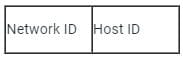Network ID + Host ID = 32 bits
Calculation
8 bit =  1 byte
× by 4 on both sides
32 bits = 4 bytes
The length of an IP address in bytes (pre-IPv6) is 4.

Test: IPv4 - Question 3

### How many bits are there in an IPv4 address?

Detailed Solution for Test: IPv4 - Question 3

There is a total of 32 bits in the IPv4 address space.
For example, if a network has the address “192.0. 2.0/24”, the number “24” refers to how many bits are contained in the network. From this, the number of bits left for address space can be calculated.
Hence the correct answer is 32.

Test: IPv4 - Question 4

There is an Ethernet port on a router were assigned an IP address of 172.16.112.1/25. Which of the following would be the valid subnet address of this host?

Detailed Solution for Test: IPv4 - Question 4

The correct option is (a)
172.16.112.0
Concept:
Subnetting is the process of creating smaller networks called subnets by taking bits from the HOST portion of an IP address.
Explanation:
Given IP IS 172.16.112.1/25 ⇒ NID (Network ID) is 25 bits.
We know that a subnet mask is obtained by replacing all NID(Net ID) bits with 1 and HID(Host ID) bits with 0.
So SM is 255.255.255.10000000 (the First 25 bits are NID and the last 7 bits are HID).
i.e, SM is 255.255.255.128.
Now perform Bitwise AND operation between subnet address and given IP address.
⇒ (255.255.255.128) and (172.16.112.1) = 172.16.112.0

Test: IPv4 - Question 5

Detailed Solution for Test: IPv4 - Question 5

Concept:

Private IP addresses are ones that only function on the local network. On the Internet, these addresses are not routable. The network router simply assigns the address to your specific device. Every device on the same network is assigned a unique private IP address.

• 192.168.0.0 - 192.168.255.255 (65,536 IP addresses)
• 172.16.0.0 - 172.31.255.255 (1,048,576 IP addresses)
• 10.0.0.0 - 10.255.255.255 (16,777,216 IP addresses)

Explanation:

• 172.30.255.255  is a valid private IP Address.
• 10.210.220.254 is a valid private IP Address.
• 172.16.21.240 is a valid private IP Address.
• 172.32.250.254 is not a valid private IP Address.

​Hence the correct answer is 3.

*Multiple options can be correct
Test: IPv4 - Question 6

which one is a valid private IP Address ?

Detailed Solution for Test: IPv4 - Question 6

Concept:

• A private IP address range is a collection of non-internet-facing IP addresses that are used in an internal network.
• Network equipment, such as routers, uses network address translation to give private IP addresses.
• IP addresses are used to identify devices on the internet or a local network.
• IP addresses also allow information to be transferred between network devices.
• 192.168.0.0 - 192.168.255.255 (65,536 IP addresses)
• 172.16.0.0 - 172.31.255.255 (1,048,576 IP addresses)
• 10.0.0.0 - 10.255.255.255 (16,777,216 IP addresses)

Hence option A and option B and option C are valid private IP Addresses.
Hence the correct answer is option A, option B, and option C.

Test: IPv4 - Question 7

An IPv4 address is a __________ address, which is categorised into different IP classes.

Detailed Solution for Test: IPv4 - Question 7

IP address is an address having information about how to reach a specific host. The 32 bit IP address is divided into five sub classes. These are:

• Class A
• Class B
• Class C
• Class D
• Class E

Class D and E are reserved for multicast and experimental purposes.

An IPv4 address is a 32 bits address, which is categorized into different IP classes.

IPv4 addresses are divided into two parts: a) Network ID b) Host ID

• Class A: This class uses 8 bits for network ID part and 24 bit for host ID.
• Class B: This class uses 16 bits for network ID and 16 bits for host ID.
• Class C: This class uses 24 bits for network ID and 8 bits for host ID.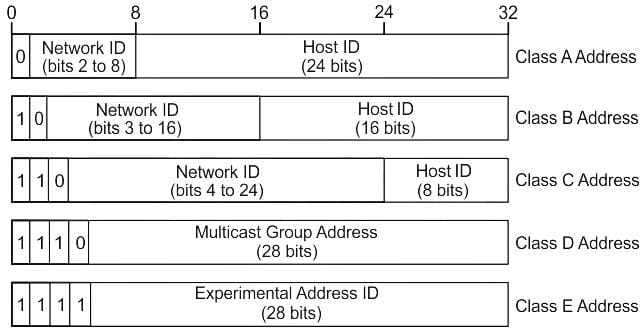Note:

• IPv6 is a128 bits address
Test: IPv4 - Question 8

An IP packet has arrived with the first 8 bits as 0100 0010. Which of the following is correct ?

Detailed Solution for Test: IPv4 - Question 8

Concept:
Minimum header size of IPv4 = 20 byte
Maximum header size of IPv4 = 60 byte
Maximum possible value (1111) = 15
The scaling factor of 60/15 = 4 is introduced

Explanation: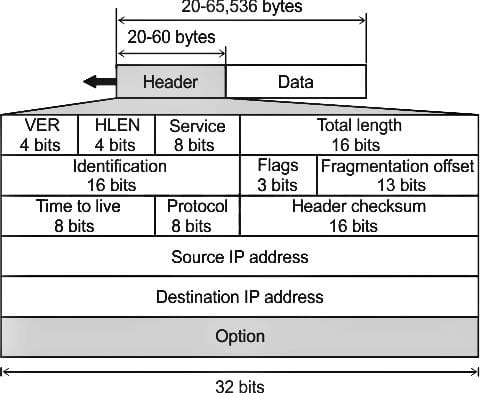But the first 8 bits in the Question are 0100 0010.
The first 4 bits indicate the version which is IPv4 (0100)
Now the next four bit indicates Header length (bits decimal value × 4) which is 2 × 4 = 8 bytes
which is not possible since the minimum header length for IPv4 is 20 bytes.
Hence Receiver will reject the packet.
Therefore option d is the correct answer.

Test: IPv4 - Question 9

Your router has the following IP address an Ethenet 0: 172.16.2.1/23. Which of the following can be valid host IDs on the LAN interface attached to the router?
1. 172.16.1.100
2. 172.16.1.198
3. 172.16.2.255
4. 172.16.3.0

Detailed Solution for Test: IPv4 - Question 9

Concept:
a.b.c.d/NID
Therefore 23 bits are in NID and only 9 bits for Host id.
Explanation:
Last two-byte of IP address is represented in binary
Ethernet: 172.16.2.1/23
Range of IP address: 172.16.00000010.0000000 to 172.16.0000011.11111111
Range of IP address: 172.16.2.0 to 172.16.3.255
First and last IP address is reserved
Valid Host IP address: 172.16.2.1 to 172.16.3.254
Statement 1: 172.16.1.100
It is not valid since it does not fall in the range
Statement 2: 172.16.1.198
It is not valid since it does not fall in the range
Statement 3: 172.16.2.255
It is valid since it does fall in the valid range
Statement 4:172.16.3.0
It is valid since it does fall in the valid range
Hence option 3 is the correct answer.
Important Point:
NID stands for network ID.

Test: IPv4 - Question 10

Match the following.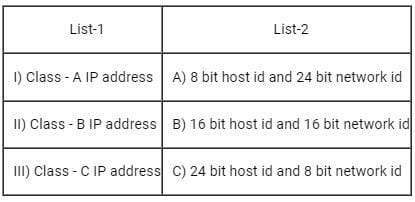Detailed Solution for Test: IPv4 - Question 10

IP address is an address having information about how to reach a specific host. The 32 bit IP address is divided into five sub classes. These are:

• Class A
• Class B
• Class C
• Class D
• Class E

Class D and E are reserved for multicast and experimental purposes.
IPv4 addresses are divided into two parts: a) Network ID b) Host ID

• Class A: This class uses 24 bits for network ID part and 8 bit for host ID.
• Class B: This class uses 16 bits for network ID and 16 bits for host ID.
• Class C: This class uses 8 bits for network ID and 16 bits for host ID.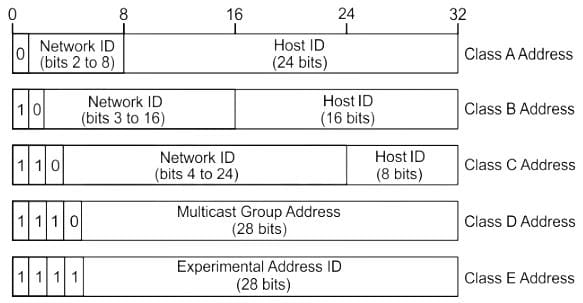## Computer Networks

12 videos|93 docs|65 tests(Scan QR code)